# Over-convergence

(diff) ← Older revision | Latest revision (diff) | Newer revision → (diff)

Convergence of a certain subsequence of partial sums of a series in a domain that is larger than the domain of convergence of the series. The following theorems on over-convergence hold:

1) If for a power series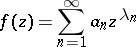with radius of convergence,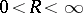, the exponentsare such that for an infinite set of values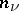of:whereis a fixed positive number, then the sequence of partial sums of orders,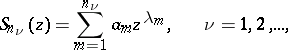converges uniformly in a sufficiently small neighbourhood of each point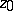of the circle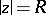on which the sum of the series foris regular.

2) If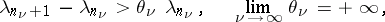then the sequence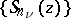converges uniformly in any closed bounded part of the domain of existence of.

The following theorem also holds (the converse of 1)): If a power series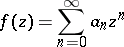with radius of convergence,, has a subsequence of partial sums that is uniformly convergent in some neighbourhood of,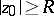, then this power series can be represented as the sum of a series with radius of convergence greater thanand a lacunary power series: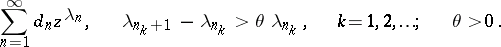The first theorem is true for many other series, in particular for Dirichlet series.

How to Cite This Entry:
Over-convergence. Encyclopedia of Mathematics. URL: http://encyclopediaofmath.org/index.php?title=Over-convergence&oldid=18212
This article was adapted from an original article by A.F. Leont'ev (originator), which appeared in Encyclopedia of Mathematics - ISBN 1402006098. See original article